# Legs

In the room are four-legged chairs, three-legged stool, and all are sitted with (one) people. I counted all the leg room and there were a total of 39. How many are there chairs, stool and people?

x =  4
y =  3
z =  7

### Step-by-step explanation:

$z=4+3=7$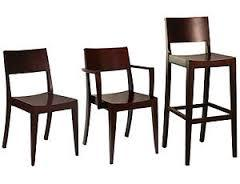We will be pleased if You send us any improvements to this math problem. Thank you!Tips to related online calculators
Do you have a system of equations and looking for calculator system of linear equations?
Do you solve Diofant problems and looking for a calculator of Diofant integer equations?

## Related math problems and questions:

• Waiting roomIn the waiting room are people and flies. Together they have 15 heads and 50 legs (fly has 6 legs). How many people and flies are in the waiting room?
• Clubhouse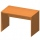There were only chairs and table in the clubhouse. Each chair had four legs, and the table was triple. Scouts came to the clubhouse. Everyone sat on their chair, two chairs were left unoccupied, and the number of legs in the room was 101. How many chairs
• Chickens and rabbitsIn the yard were chickens and rabbits. Together they had 18 heads and 56 legs. How many chickens and how many rabbits were in the yard?
• Chairs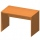In the two dining rooms in the recreational building, there are equally arranged chairs around the tables. A maximum of 78 people can dine in the first dining room and 54 people in the second. How many chairs can be around one table?
• Diofant equationIn the set of integers (Z) solve the equation: ? Write result with integer parameter ? (parameter t = ...-2,-1,0,1,2,3... if equation has infinitely many solutions)
• Two integers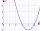Two integers, a and b, have a product of 36. What is the least possible sum of a and b?
• Diofant 2Is equation ? solvable on the set of integers Z?
• Four integersFnd four consecutive integers so that the product of the first two is 70 times smaller than the product of the next two.
• LegsCancer has 5 pairs of legs. The insect has 6 legs. 60 animals have a total of 500 legs. How much more are cancers than insects?
• RectangleThe perimeter of the rectangle is 22 cm and content area 30 cm2. Determine its dimensions, if the length of the sides of the rectangle in centimeters is expressed by integers.
• The manila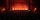The manila theater charged ticket prices as follow: adults php500, student php250, and children below 12 is php100. The theater collected a total of php100,000.00 from an audience of 250 people. What is a possible composition of the audience regarding the
• LineStraight-line passing through points A [-3; 22] and B [33; -2]. Determine the total number of points of the line in which both coordinates are positive integers.
• Heads and legs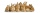108 legs and 33 heads how many chickens. How many rabbits are there?
• Two numbers 7The sum of two consecutive even numbers is 30. Find the numbers.
• AddingDivide number 135 into two additions so that one adds 30 more than 2/5 of the other add. Write the bigger one.
• There 5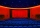There were 429 people at the play. Admission was 50 pesos each for adults and 35 pesos each for children. The receipts were 17265 pesos. How many adults and how many children attended?
• Dining roomThe dining room has 11 tables (six and eight seats). In total there are 78 seats in the dining room. How many are six-and eight-seat tables?